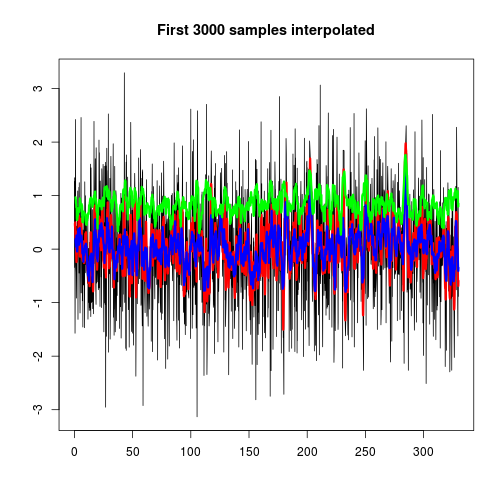# An Introduction to rroad Package

``````road_len_m <- 1000          # road length
speed_kmh <- 80             # car speed in km/h
sample_rate_hz <- 200       # sampling rate of the 3D accelerometer
speed_ms <- speed_kmh / 3.6 # car speed in m/s
sample_len <- round(speed_ms / sample_rate_hz, digits = 2) # sample size
num_samples <- round(road_len_m / sample_len) # how many samples we collected
GRAVITY_ACCEL <- 9.80665    # ms^-2

print(sample_len)
``````
``````##  0.11
``````
``````print(num_samples)
``````
``````##  9091
``````

First, we use some sample data obrained from a 3D-accelerometer. We need to trim NA valued from the signal, because there might be gaps (NA values) and the interpolation doesn't work with NAs.

``````# random signal with simulated gravity
random_accz <- (rnorm(num_samples) - GRAVITY_ACCEL)

# random gaps in the signal to later demonstrate gaps interpolation
random_gaps <- sapply(rnorm(num_samples),
function(x){ifelse(abs(x) > .7, 1, NA)})

signal <- data.frame(
sampleid = seq_len(num_samples),
dist_meters = seq(from = 0, to = road_len_m, by = sample_len),
accZ = random_accz * random_gaps
)

plot(signal\$dist_meters, signal\$accZ, type = "o", pch = "+", cex = .5,
main = "Accelerometer signal (original)",
xlab = "Distance traveled [m]",
ylab = expression( paste("Z-acceleration [", m * s ^ -2, "]") ))
``````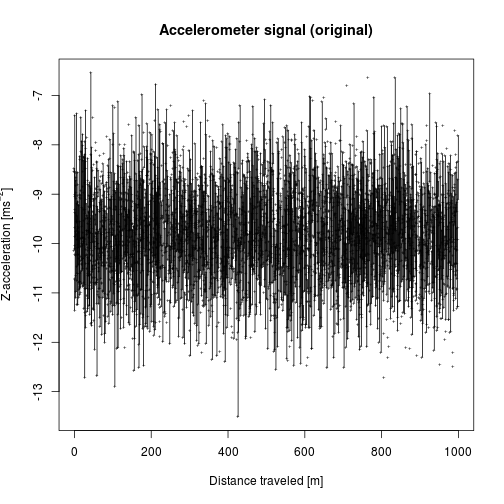``````# signal without the standard gravity acceleration
(signal\$accZ_orig - GRAVITY_ACCEL) -> signal\$accZ_nogravity

# z-score normalization (subtracting mean and dividing by sd)
scale(signal\$accZ_orig) -> signal\$accZ

# removing leading and trailing NAs in the whole matrix
na.trim(signal) -> signal

plot(signal\$dist_meters, signal\$accZ, type = "o", pch = "+", cex = .5,
main = "Accelerometer signal (z-score normalized)",
xlab = "Distance traveled [m]",
ylab = expression( paste("Z-acceleration [", m * s ^ -2, "]") ))
``````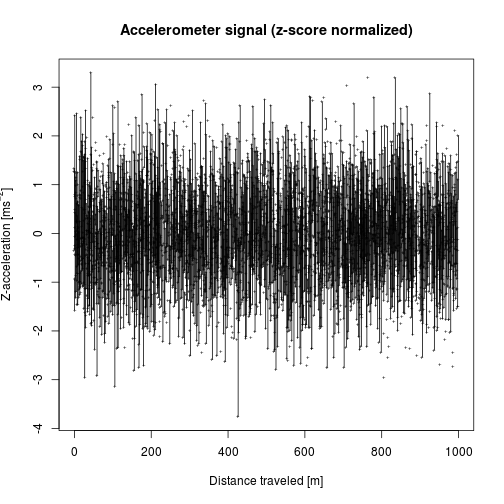Now, we look at the gaps closely:

``````head(signal, 500) -> signal_head
type = "o", xlab = NA, ylab = NA, pch = "+", cex = .5,
main = paste("First", nrow(signal_head), "samples with gaps"))
``````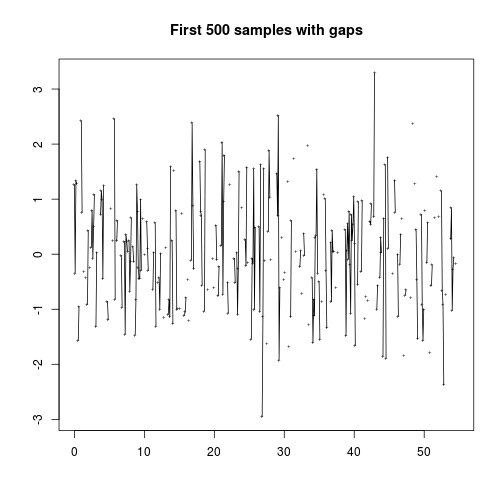We need to interpolate the values between the gaps:

``````na.approx(signal\$accZ, na.rm = FALSE) -> signal\$accZ_approx

type = "p", pch = "+", cex = .5, xlab = NA, ylab = NA,
main = paste("First", nrow(signal_head), "samples interpolated"))

col = "red", pch = ".", xlab = NA, ylab = NA)
``````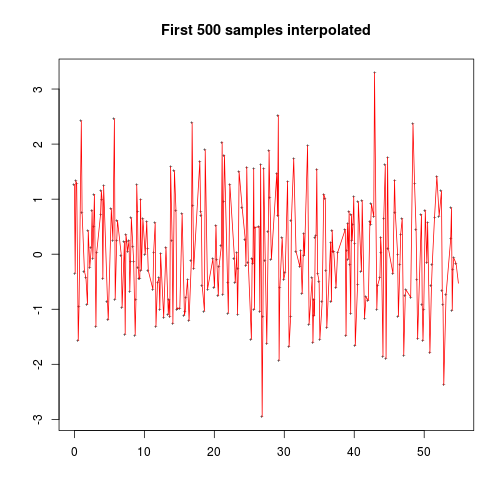We can also analyze frequency content of the signal by using Continuous Wavelet Transform (CWT). The following plot is called “scaleogram”.

``````w <- wt(cbind(signal\$sampleid, signal\$accZ_approx), dj = 1/2)
plot(w)
``````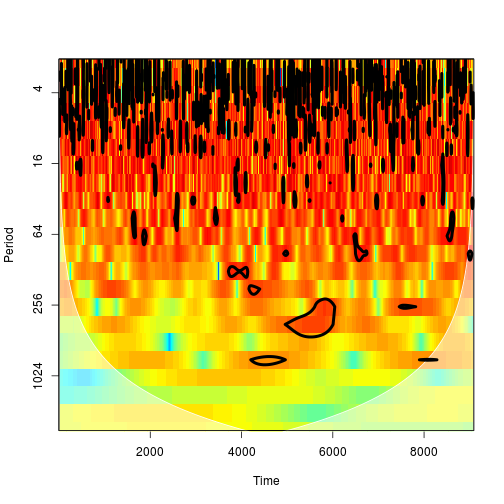We can extract the CWT coeficients representing certaing frequency bands. The `power.corr` matrix represents bias-correction version.

``````nscales <- nrow(w\$power.corr)
signal\$cwt_mid  <- w\$power.corr[floor(.5 * nscales),]
signal\$cwt_high <- w\$power.corr[floor(.2 * nscales),]
signal\$cwt_low  <- w\$power.corr[floor(.8 * nscales),]

plot(signal\$cwt_high, type = "l")
lines(signal\$cwt_mid, col = "blue", lw = 4)
lines(signal\$cwt_low, col = "red", lw = 4)
``````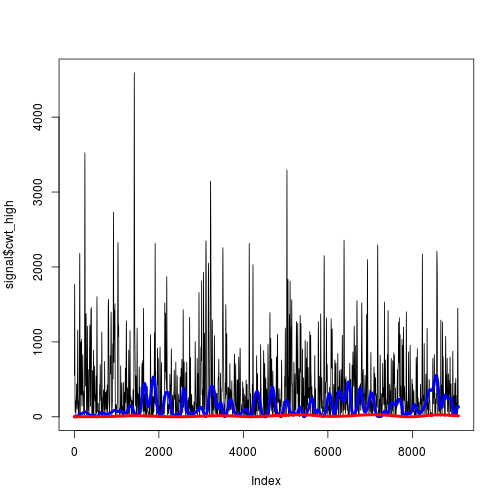Here, we compute moving average and root mean squared value:

``````rollmean(signal\$accZ_approx, k = 10, fill = NA) -> signal\$rollmean10
rollmean(signal\$accZ_approx, k = 20, fill = NA) -> signal\$rollmean20

rms <- function(x) sqrt(mean(x^2)) # same as `rms` from `seewave` package
rollapply(signal\$accZ_approx, width = 20, fill = NA, FUN = rms ) -> signal\$rms20
``````
``````head(signal, 3000) -> signal_head